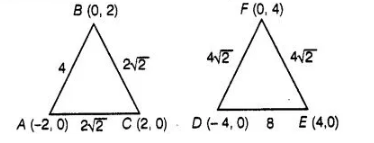# Δ ABC with vertices A(0 ,- 2,0), B(2, 0)

Question:

Δ ABC with vertices A(0 ,- 2,0), B(2, 0) and C(0,2) is similar to ΔDEF with vertices D (- 4, 0), E(4, 0) and F(0, 4).

Solution:

True

$\therefore$ Distance between $A(2,0)$ and $B(2,0), A B=\sqrt{[2-(2)]^{2}+(0-0)^{2}}=4$

$\left[\because\right.$ distance between the points $\left(x_{1}, y_{1}\right)$ and $\left.\left(x_{2}, y_{2}\right), d=\sqrt{\left(x_{2}-x_{1}\right)^{2}+\left(y_{2}-y_{1}\right)^{2}}\right]$

Similarly, distance between $B(2,0)$ and $C(0,2), B C=\sqrt{(0-2)^{2}+(2-0)^{2}}=\sqrt{4+4}=2 \sqrt{2}$

In $\triangle A B C$, distance between $C(0,2)$ and $A-(2,0)$,

$C A=\sqrt{\left[0-(2)^{2}\right]+(2-0)^{2}}=\sqrt{4+4}=2 \sqrt{2}$

Distance between $F(0,4)$ and $D(-4,0), F D=\sqrt{(0+4)^{2}+(0-4)^{2}}=\sqrt{4^{2}+(-4)^{2}}=4 \sqrt{2}$

Distance between $F(0,4)$ and $E(4,0), F E=\sqrt{(4-0)^{2}+(0-4)^{2}}=\sqrt{4^{2}+4^{2}}=4 \sqrt{2}$

and distance between $E(4,0)$ and $D(-4,0), E D=\sqrt{[4-(-4)]^{2}+(0)^{2}}=\sqrt{8^{2}}=8$

Now, $\quad \frac{A B}{O E}=\frac{4}{8}=\frac{1}{2}, \frac{A C}{D F}=\frac{2 \sqrt{2}}{4 \sqrt{2}}=\frac{1}{2}, \frac{B C}{E F}=\frac{2 \sqrt{2}}{4 \sqrt{2}}=\frac{1}{2}$

$\therefore$ $\frac{A B}{D E}=\frac{A C}{D F}=\frac{B C}{E F}$

Here, we see that sides of $\triangle A B C$ and $\triangle F D E$ are propotional.Hence, both the triangles are similar.                                                                                                                                [by SSS rule]